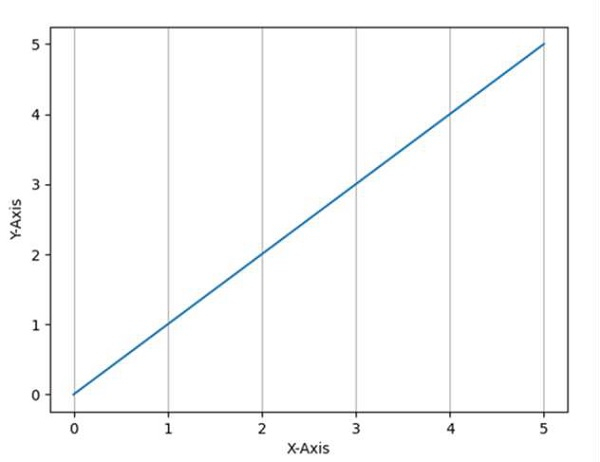# Getting vertical gridlines to appear in line plot in matplotlib

Using plt.grid(axis="x") method, we can plot vertical gridlines.

## Steps

• Make a list of numbers.

• Set the X-axis label using plt.xlabel() method.

• Set the Y-axis label using plt.ylabel() method.

• Toggle the gridlines, and optionally set the properties of the lines, using plt.grid() method.

• To show the figure, use the plt.show() method, where the argument axis can be “x”, “y” or “both”.

## Example

from matplotlib import pyplot as plt

plt.plot([0, 5], [0, 5])

plt.ylabel("Y-axis ")
plt.xlabel("X-axis ")

plt.grid(axis="x")

plt.show()

## Output